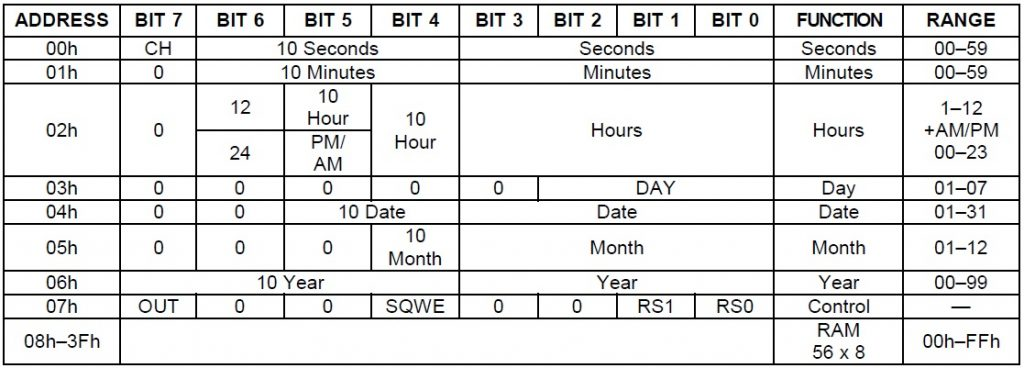# DS1307 RTC with PIC Microcontroller

Home Forums Microcontrollers PIC Microcontroller DS1307 RTC with PIC Microcontroller

Viewing 2 posts - 1 through 2 (of 2 total)
• Author
Posts
• #11510

With reference to the article Digital Clock using PIC Microcontroller and DS1307 RTC. Can anyone help me with the explanation of setting hour in the switch case 1 ?

```// LCD module connections
sbit LCD_RS at RB2_bit;
sbit LCD_EN at RB3_bit;
sbit LCD_D4 at RB4_bit;
sbit LCD_D5 at RB5_bit;
sbit LCD_D6 at RB6_bit;
sbit LCD_D7 at RB7_bit;
sbit LCD_RS_Direction at TRISB2_bit;
sbit LCD_EN_Direction at TRISB3_bit;
sbit LCD_D4_Direction at TRISB4_bit;
sbit LCD_D5_Direction at TRISB5_bit;
sbit LCD_D6_Direction at TRISB6_bit;
sbit LCD_D7_Direction at TRISB7_bit;
// End LCD module connections

unsigned short read_ds1307(unsigned short address)
{
unsigned short r_data;
I2C1_Start();
I2C1_Wr(0xD0); //address 0x68 followed by direction bit (0 for write, 1 for read) 0x68 followed by 0 --> 0xD0
I2C1_Wr(address);
I2C1_Repeated_Start();
I2C1_Wr(0xD1); //0x68 followed by 1 --> 0xD1
r_data=I2C1_Rd(0);
I2C1_Stop();
return(r_data);
}

void write_ds1307(unsigned short address,unsigned short w_data)
{
I2C1_Start(); // issue I2C start signal
//address 0x68 followed by direction bit (0 for write, 1 for read) 0x68 followed by 0 --> 0xD0
I2C1_Wr(0xD0); // send byte via I2C (device address + W)
I2C1_Wr(address); // send byte (address of DS1307 location)
I2C1_Wr(w_data); // send data (data to be written)
I2C1_Stop(); // issue I2C stop signal
}

unsigned char BCD2UpperCh(unsigned char bcd)
{
return ((bcd >> 4) + '0');
}

unsigned char BCD2LowerCh(unsigned char bcd)
{
return ((bcd & 0x0F) + '0');
}

int Binary2BCD(int a)
{
int t1, t2;
t1 = a%10;
t1 = t1 & 0x0F;
a = a/10;
t2 = a%10;
t2 = 0x0F & t2;
t2 = t2 << 4;
t2 = 0xF0 & t2;
t1 = t1 | t2;
return t1;
}

int BCD2Binary(int a)
{
int r,t;
t = a & 0x0F;
r = t;
a = 0xF0 & a;
t = a >> 4;
t = 0x0F & t;
r = t*10 + r;
return r;
}

int second;
int minute;
int hour;
int hr;
int day;
int dday;
int month;
int year;
int ap;

unsigned short set_count = 0;
short set;

char time[] = "00:00:00 PM";
char date[] = "00-00-00";

void main()
{
I2C1_Init(100000); //DS1307 I2C is running at 100KHz
CMCON = 0x07;   // To turn off comparators
ADCON1 = 0x06;  // To turn off analog to digital converters
TRISA = 0x07;
PORTA = 0x00;
Lcd_Init();                        // Initialize LCD
Lcd_Cmd(_LCD_CLEAR);               // Clear display
// Lcd_Cmd(_LCD_CURSOR_OFF);          // Cursor off
Lcd_Cmd(_LCD_BLINK_CURSOR_ON);
Lcd_out(1,1,"Time:");
Lcd_out(2,1,"Date:");

do
{
set = 0;
if(PORTA.F0 == 0)
{
Delay_ms(100);
if(PORTA.F0 == 0)
{
set_count++;
if(set_count >= 7)
{
set_count = 0;
}
}
}
if(set_count)
{
if(PORTA.F1 == 0)
{
Delay_ms(100);
if(PORTA.F1 == 0)
set = 1;
}
if(PORTA.F2 == 0)
{
Delay_ms(100);
if(PORTA.F2 == 0)
set = -1;
}
if(set_count && set)
{
switch(set_count)
{
case 1:
hour = BCD2Binary(hour);
hour = hour + set;
hour = Binary2BCD(hour);
if((hour & 0x1F) >= 0x13)
{
hour = hour & 0b11100001;
hour = hour ^ 0x20;
}
else if((hour & 0x1F) <= 0x00)
{
hour = hour | 0b00010010;
hour = hour ^ 0x20;
}
write_ds1307(2, hour); //write hour
break;
case 2:
minute = BCD2Binary(minute);
minute = minute + set;
if(minute >= 60)
minute = 0;
if(minute < 0)
minute = 59;
minute = Binary2BCD(minute);
write_ds1307(1, minute); //write min
break;
case 3:
if(abs(set))
write_ds1307(0,0x00); //Reset second to 0 sec. and start Oscillator
break;
case 4:
day = BCD2Binary(day);
day = day + set;
day = Binary2BCD(day);
if(day >= 0x32)
day = 1;
if(day <= 0)
day = 0x31;
write_ds1307(4, day); // write date 17
break;
case 5:
month = BCD2Binary(month);
month = month + set;
month = Binary2BCD(month);
if(month > 0x12)
month = 1;
if(month <= 0)
month = 0x12;
write_ds1307(5,month); // write month 6 June
break;
case 6:
year = BCD2Binary(year);
year = year + set;
year = Binary2BCD(year);
if(year <= -1)
year = 0x99;
if(year >= 0x50)
year = 0;
write_ds1307(6, year); // write year
break;
}

}
}

second = read_ds1307(0);
minute = read_ds1307(1);
hour = read_ds1307(2);
hr = hour & 0b00011111;
ap = hour & 0b00100000;
dday = read_ds1307(3);
day = read_ds1307(4);
month = read_ds1307(5);
year = read_ds1307(6);

time = BCD2UpperCh(hr);
time = BCD2LowerCh(hr);
time = BCD2UpperCh(minute);
time = BCD2LowerCh(minute);
time = BCD2UpperCh(second);
time = BCD2LowerCh(second);

date = BCD2UpperCh(day);
date = BCD2LowerCh(day);
date = BCD2UpperCh(month);
date = BCD2LowerCh(month);
date = BCD2UpperCh(year);
date = BCD2LowerCh(year);

if(ap)
{
time = 'P';
time = 'M';
}
else
{
time = 'A';
time = 'M';
}
Lcd_out(1, 6, time);
Lcd_out(2, 6, date);
Delay_ms(100);
}while(1);
}
```
#11519

For understanding those HOUR setting steps, you should read the datasheet of DS1307.

You can find that all bits of HOUR registers are not used for indicating hour magnitude. It can indicate in 24 or 12 hour mode.Viewing 2 posts - 1 through 2 (of 2 total)

You must be logged in to reply to this topic.